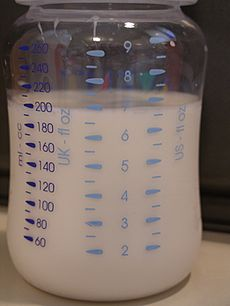# System of units facts for kids

Kids Encyclopedia FactsA baby bottle that measures in three measurement systems—imperial (UK), US customary, and metric.

A system of units is a collection of units of measurement. The quantities described by the units are related to each other by specific rules. These rules may not contradict each other. An example of a system like this is one needed for the problems of the economic system.

## Base and derived units

Some of the units are called "base units". These units can be defined by arbitrarily. Other units are called derived units. They are defined using the base units. The most common systems of units today are the International System of Units (SI), the Imperial units and the United States customary units.

The base units of SI are the metre, the second, the kilogram, the ampere, the kelvin, the candela and the mole. SI has many derived units which include the watt, newton and joule. SI is called a "coherent system of units" because the constant factor in the definition of it derived units is always 1.

The base units of the imperial system and the United States customary system are the pound avoirdupois, the yard and the second. Derived units found in the Imperial and Customary systems of units include horsepower and Pounds per square inch.

## Coherent units

The relationship force, energy and power is an example of coherence. The equations are

force = kf × mass × acceleration
energy = ke × force × distance
power = kp × energy / time.
If kf = 1 and ke = 1 and kp = 1 then the relationships are coherent.

In SI, the equations become

newtons = kilograms × metres per second squared
joules = newtons × metres
watts = joules / second.
SI is therefore a coherent system.

In the cgs system of units, the equations become

dynes = grams × centimetres per second squared
ergs = dynes × centimetres
ergs per second = erg / second.
The equations for the cgs system do not have any additional constants. It is therefore a coherent system.

In the MKS-gravitational system, the equations become

kilogram-force = kilograms × metres per second squared / 9.80665
Pferdestärke = 75 × kilogram-force × metres /seconds
The equations in the MKS-gravitational system have factors 75 and 9.80655. The system is therefore not is therefore not a coherent.System of units Facts for Kids. Kiddle Encyclopedia.